Translating to and from Word Statements

 Problem Set 1 Problem Set 2 Problem Set 3Select a problem set using the buttons above, then use your mouse or tab key to select a question. Fill in the blank with the correct answer for each problem. When you have answered all of the questions, ask Charlie how you did.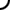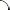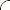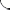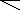Translate each word statement to a mathematical expression. Use '*' for multiplication and '/' for division. Example: 'The product of four and seven.' 4*7 Example: 'The quotient of four and seven.' 4/7   The product of two and six. The quotient of x and five. The product of y and three.   Finish translating each mathematical expression into words. Fill in each blank with product or quotient.   8 • 7 The of eight and seven. 6 ÷ 2 The of six and two. 20 ÷ 5 The of twenty and five.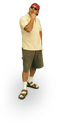Translate each word statement to a mathematical expression. Use '*' for multiplication and '/' for division. Example: 'The product of four and seven.' 4*7 Example: 'The quotient of four and seven.' 4/7   The quotient of sixteen and two. The product of eleven and two. The quotient of thirty and fifteen.   Finish translating each mathematical expression into words. Fill in each blank with product or quotient.   5 • 10 The of five and ten. 12 ÷ 4 The of twelve and four. 4 • 2 The of four and two.Translate each word statement to a mathematical expression. Use '*' for multiplication and '/' for division. Example: 'The product of four and seven.' 4*7 Example: 'The quotient of four and seven.' 4/7   The quotient of nine and three. The quotient of eight and four. The product of five and seven.   Finish translating each mathematical expression into words. Fill in each blank with product or quotient.   15 ÷ 3 The of fifteen and three. 3 • 7 The of three and seven. 13 • 1 The of thirteen and one.©  2009  LarryPerezWeb Design:  Patrick Quigley